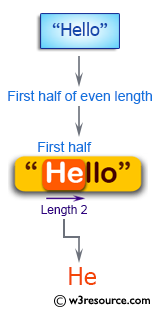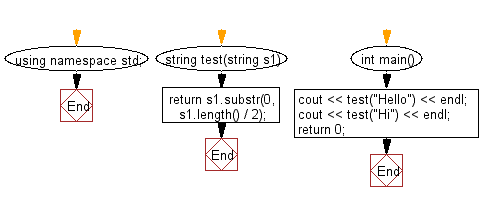﻿ C++ : New string of the 1st half of a string of even length# C++ Exercises: Create a new string of the first half of a given string of even length

## C++ Basic Algorithm: Exercise-61 with Solution

Write a C++ program to create a new string of the first half of a given string of even length.

Sample Solution:

C++ Code :

``````#include <iostream>
using namespace std;

string test(string s1)
{
return s1.substr(0, s1.length() / 2);
}

int main()
{
cout << test("Hello") << endl;
cout << test("Hi") << endl;
return 0;
}
``````

Sample Output:

```He
H
```

Pictorial Presentation:Flowchart:C++ Code Editor:

Contribute your code and comments through Disqus.

What is the difficulty level of this exercise?

﻿